Published: 19 October 2018

# Adaptive vibration control for a cantilevered beam using actuating and sensing functions of a piezoelectric bimorph

Ruiping Wang1
Ting Zhang2
Yue Yang3
1, 2, 3College of Mechanical Engineering, Shanghai University of Engineering Science, Shanghai, People’s Republic of China
Corresponding Author:
Ting Zhang
Views 108

#### Abstract

The paper investigates that the free vibration for a smart cantilevered beam utilizing actuating and sensing properties of a piezoelectric bimorph is suppressed by a adaptive controller. Through the bimorph, the suppression vibration of a flexible cantilevered beam is studied. Because of uncertain parameters in the dynamic model, a adaptive control is adopted with a minimum variance self-tuning direct regulator to control the free vibration of the smart beam. By some simulation and experiment verifications, the adaptive control is proved to be feasible for attenuating the free vibration of the smart beam.

## 1. Introduction

A piezoelectric bimorph, which is consisted of two pieces of piezoelectric patches bonded symmetrically on the top and bottom of a copper electrode beam , has been widely used as electroacoustic transducer, energy harvester , micropump driver  and microrobot for its sensitive response characteristics. If the piezoelectric patch is applied with the actuating voltages, one active piezoelectric layer contracts and the other expands simultaneously, thus the bimorph bends . In sensing applications, bending the bimorph may produce a voltage which can be used to measure displacement or acceleration of a space structure. A self-sensing piezoelectric pump with a bimorph transducer is presented to realize the functions of fluid transportation and output flow and pressure self-testing simultaneously through only a single piezoelectric element . Therefore, utilizing a piezoelectric bimorph for concurrent sensing and actuating functions, it is conventional to attenuate free vibration for a cantilevered beam.

For suppressing vibration of a smart beam, adopting the adaptive control attaches much attention from many researchers in recent years. Adaptive control is the control method that adapts itself to a controlled system under such changing conditions, uncertain external environment or unknown physical performance parameters. A digital control of a smart piezoelectric beam is considered to achieve vibration regulation under parametric uncertainties in the model parameters (damping ratios, frequencies, levels of non linearities and cross coupling, control input parameters) . However, in the paper, vibration suppression of a cantilevered beam with a piezoelectric bimorph with uncertain parameters in the dynamic model is investigated with a adaptive control law. A simple and effective control method is required to achieve damping free vibration of the flexible cantilever beam in a shorter time, and the multi-degree-freedom coupling vibration problems of a smart beam with distributed sensors and actuators  further are studied in the future. It is obvious that a hybrid control based on a complex dynamic model isn’t easily implemented in order to run slowly with lengthy program in computer especially for a multi-degree-freedom system. Therefore, a simple adaptive control is adopted with a minimum variance self-tuning direct regulator  as fundamental exploration to suppress vibration of a flexible beam with piezoelectric bimorphs.

The rest of this paper is organized as follows: In Section 2, the actuating and sensing properties of the piezoelectric bimorph is introduced. In Section 3, a minimum variance self-tuning direct regulator to suppress vibration for the smart beam is designed and some simulation and experiment verifications are given. Finally, some conclusions are shown in Section 4.

## 2. Adaptive control design for a smart beam

Fig. 1 shows a cantilever beam bonded with a piezoelectric bimorph, and the actuating layer is applied with a actuating voltage by the HPV power amplifier. The data acquisition device is used to collect response voltage from the sensing layer of the bimorph. in the paper, an adaptive controller is adopted to suppress the free vibration of the cantilever beam which cannot get their mechanical property parameters easy.

Fig. 1A cantilevered beam with a piezoelectric bimorph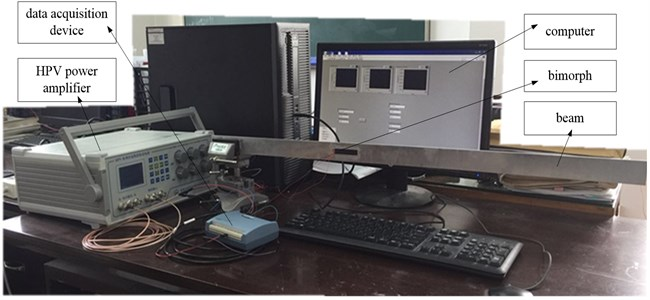In the paper, an adaptive controller is adopted to suppress the free vibration of the cantilever beam. the minimum variance self-tuning control  is one kind of adaptive control laws, it is described as follows.

First, the CARMA model is given as:

1
$a\left({z}^{-1}\right)Y\left(k\right)=b\left({z}^{-1}\right)U\left(k-d\right)+c\left({z}^{-1}\right)\xi \left(k\right),$

where $U\left(k\right)$ and $Y\left(k\right)$ are the input and output of the system respectively, $d$ is pure time delay, $\mathrm{\xi }\left(k\right)$ is the random disturbance, $k$ is sample point and:

$\left\{\begin{array}{l}a\left({z}^{-1}\right)=1+{a}_{1}{z}^{-1}+{a}_{2}{z}^{-2}+\cdots +{a}_{{n}_{a}}{z}^{-{n}_{a}},\\ b\left({z}^{-1}\right)={b}_{0}+{b}_{1}{z}^{-1}+{b}_{2}{z}^{-2}+\cdots +{b}_{{n}_{b}}{z}^{-{n}_{b}}\begin{array}{ll},\left({b}_{0}\ne 0\right),& \end{array}\\ c\left({z}^{-1}\right)=1+{c}_{1}{z}^{-1}+{c}_{2}{z}^{-2}+\cdots +{c}_{{n}_{c}}{z}^{-{n}_{c}},\end{array}\right\$

when the optimal output of system in sample point $k+d$ is assumed as ${Y}^{\mathrm{*}}\left(k+d|k\right)$, the control strategy $U$  is expressed as:

2
$f\left({z}^{-1}\right)U\left(k\right)=c\left({z}^{-1}\right){Y}^{\mathrm{*}}\left(k+d\right)-g\left({z}^{-1}\right)Y\left(k\right),$

where:

$\left\{\begin{array}{l}g\left({z}^{-1}\right)={g}_{0}+{g}_{1}{z}^{-1}+\cdots +{g}_{{n}_{g}}{z}^{-{n}_{g}}\begin{array}{ll},\left({n}_{g}={n}_{a}-1\right),& \end{array}\\ f\left({z}^{-1}\right)={f}_{0}+{f}_{1}{z}^{-1}+\cdots +{f}_{{n}_{f}}{z}^{-{n}_{f}}\begin{array}{ll},\left({n}_{f}={n}_{b}+d-1\right).& \end{array}\end{array}\right\$

## 3. Simulation and experiment verifications

The simulation is implemented in MatLab, and some corresponding parameters of the CARMA model are given with $a=$ [1 –01 0.936], $b=$ [0.006 –0.006] and $c=$ [1 0.006] through online identification by recursive extended least square method. when the adaptive controller is running, the control voltage and the control result are shown in Fig. 2 and Fig. 3 respectively. From the control result in Fig. 3, the free vibration of the smart beam with control is damped more quickly in a shorter time compared with that at no control.

Fig. 2A simulation control voltage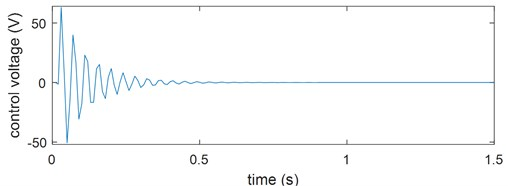Fig. 3A simulation control result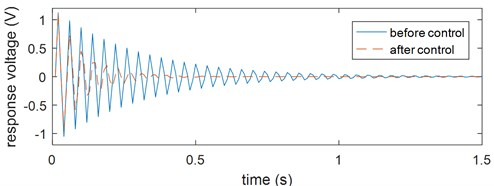Fig. 4An experiment control voltage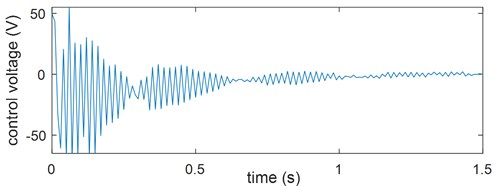Fig. 5An experiment control result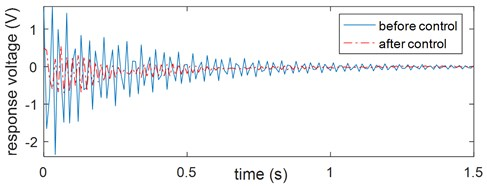The experimental verification is implemented in LabVIEW shown in Fig. 1. When the tip end of the smart beam suffers from an external disturbance, the sensing layer of the bimorph generates a response voltage which is sent to the computer by the data acquisition device. Then, through the adaptive control law, a control voltage is calculated, is outputted to the DA port of the data acquisition device and is applied on the actuating layer of the bimorph bonded on the beam after amplifying by the HPV power amplifier. The control voltage and control result from experiment are shown in Fig. 4 and Fig. 5 respectively. From the result after control, the free vibration of the smart beam is proved to be suppressed effectively with the adaptive controller compared with that before control.

## 4. Conclusions

In the paper, a piezoelectric bimorph as an integration of sensor and actuator is adapted to suppress free vibration for a cantilevered beam. when some parameters in the dynamic model of the smart beam are uncertain, an adaptive control law is employed to regulate the free the vibration with a minimum variance self-tuning direct regulator. Thought some simulations and experiments, the adaptive controller is verified to be useful for vibration control. It is fundamental to explore the research of multi-degree-of-freedom coupling vibration of smart structure with distributed sensors and actuators.

31 July 2018
Accepted
12 August 2018
Published
19 October 2018
SUBJECTS
Vibration generation and control
Keywords
piezoelectric bimorph
vibration suppression